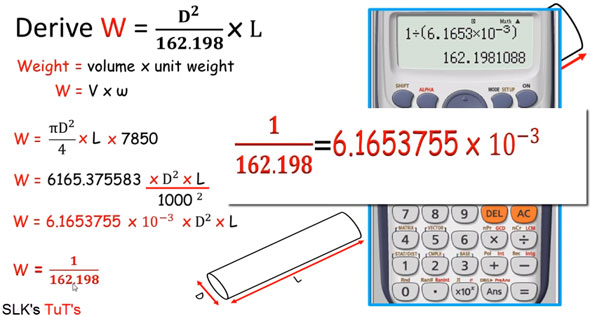# How to estimate the weight of a round shaped steel barIn this construction video tutorial, you will learn the detailed method for estimating the weight of mild steel round bar with the plug chugging formula.

The formula is represented as W = D2/162.198xL
This formula is very useful in determining the weight of mild steel bar in Kg.

Here, D denotes dia of bar in mm and L denotes length of bar in meters.

In this video, a round shape cross section bar is selected and it?s length is taken as L and diameter is D.

Weight = Volume x Unit Weight

W = V x ? (Volume = X-section area x length and omega is the unit weight of material)

As the bar is round, the cross sectional are will be pD2/4

So, putting the value we get the following:-

W = pD2/4 x L

For mild steel, unit weight is taken as 7850 kg/m3 or 222.32 kg/ft3

Putting the value, we get W = pD2/4 x L

= pD2/4 x L x 7850 = 6165.375583 x D2 x L/10002

= 6165.3755 x 10-3 x D2 x L

= 1/162.198 x D2 x L

So, W = D2/162.198 x L

To get more details, watch the following video tutorial.

Video Source: SL Khan